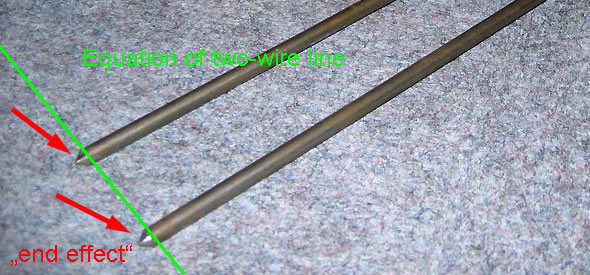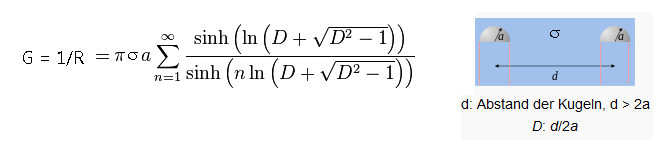# Approximation of the fringing effect

## Motivation

To measure the ground conductivity and relative permittivity with high frequency using an open wire line (OWL) you need to know about the behaviour of the end of the two-wire line:

## Theory

The form of the end of the two wire line are two cylinder cones by sharpening. This form is approximated here by two hemispheres.Picture: End of open two-wire line.

If you remove one half of the hemisphere at the equation "capacitance of two spheres, equal radius"  you get the capacity of the end of the open line:By an substitution of the geometry in both general basic equations (capacity and resistance) you get the conductance of the end of the two-wire line:Conductance and capacity are parallel.

 Wikipedia: "Capacitance"